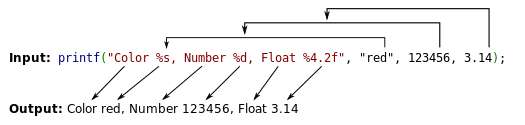# Format string vulnerability principle introduction¶

First, a brief introduction to the principles of formatting string vulnerabilities.

## Format string function introduction¶

The format string function accepts a variable number of arguments and uses the first argument as a format string, from which the parsed argument is parsed. In general, formatting a string function is to convert the data represented in the computer's memory into our human-readable string format. Almost all C/C++ programs use formatted string functions to output information, debug programs, or process strings. In general, formatted strings are mainly divided into three parts when utilized.

• Format string function
• format string
• Subsequent parameters, optional

Here we give a simple example, I believe that most people have been exposed to printf functions and the like. Then we will introduce them one by one.### Format string function¶

Common formatted string functions have

• Enter

• scanf
• output

Function Basic introduction

| printf | output to stdout | | fprintf | Output to the specified FILE stream | | vprintf | Formatting output to stdout based on parameter list | | vfprintf | Formatting output to the specified FILE stream according to the parameter list | | sprintf | Output to String | | snprintf | Output the specified number of bytes to the string | | vsprintf | Formatting output to a string based on a parameter list | | vsnprintf | Formatting Output Specified Bytes to Strings Based on Parameter List | | setproctitle | set argv | | syslog | output log | | err, verr, warn, vwarn 等 | ... |

### Format string¶

Here we understand the format of the format string, the basic format is as follows

%[parameter][flags][field width][.precision][length]type


For the meaning of each pattern, please refer to the Wikipedia [format string] (https://zh.wikipedia.org/wiki/%E6%A0%BC%E5%BC%8F%E5%8C%96% E5%AD%97%E7%AC%A6%E4%B8%B2). The corresponding choices in the following patterns need to focus on

• parameter

• n\$, get the specified parameters in the format string

• flag

• field width

• the minimum width of the output

• precision

• the maximum length of the output

• length, the length of the output
• hh, output one byte
• h, output a double byte
• type

• d/i, signed integer

• u, unsigned integer
• x/X, hexadecimal unsigned int. x uses lowercase letters; X uses uppercase letters. If the precision is specified, the number of the output is zero when it is insufficient. The default precision is 1. When the precision is 0 and the value is 0, the output is empty.
• o, octal unsigned int. If the precision is specified, the number of the output is zero when it is insufficient. The default precision is 1. When the precision is 0 and the value is 0, the output is empty.
• s, if the l flag is not used, the null end string is output until the upper limit specified by the precision; if no precision is specified, all bytes are output. If the l flag is used, the corresponding function parameter points to an array of type wchar_t, and each wide character is converted to a multi-byte character at the time of output, which is equivalent to calling the wcrtomb function.
• c, if the l flag is not used, convert the int parameter to unsigned char output; if the l flag is used, the wint_t parameter is converted to a wchart_t array containing two elements, the first element containing the character to be output The second element is a null wide character.
• p, void * type, output the value of the corresponding variable. Printf("%p",a) prints the value of the variable a in the format of the address, printf("%p", &a) prints the address where the variable a is located.
• n, does not output characters, but writes the number of characters that have been successfully output to the variable pointed to by the corresponding integer pointer parameter.
• %, '%' literal, does not accept any flags, width.

### Parameters¶

It is the corresponding variable to be output.

## Format string vulnerability principle¶

In the beginning, we will give a basic introduction to formatting strings, and here are some more detailed content. We said above that the format string function is parsed according to the format string function. Then the corresponding number of parameters to be parsed is naturally controlled by this format string. For example, '%s' indicates that we will output a string argument.

Let’s continue with the above example as an example.

![Basic example] (./figure/printf.png)

For such an example, before entering the printf function (that is, printf has not been called yet), the layout on the stack from high address to low address is as follows

some value

3.14

123456

addr of format string: Color %s...


Note: Here we assume that the value above 3.14 is some unknown value.

After entering printf, the function first gets the first parameter, and one by one reads two characters.

• The current character is not % and is output directly to the corresponding standard output.
• the current character is %, continue reading the next character
• If there are no characters, an error is reported.
• If the next character is %, output %
• Otherwise, according to the corresponding characters, get the corresponding parameters, parse and output them

So suppose that at this time we wrote the following when we wrote the program.

printf("Color %s, Number %d, Float %4.2f");


At this point we can see that we did not provide the parameters, then how will the program run? The program will still run, parsing the three variables above the formatted string address on the stack into

1. Parse the string corresponding to its address
2. Parse the integer value corresponding to its content
3. Parse the floating point value corresponding to its content

For 2, 3, it doesn't matter, but for 1, if an inaccessible address, such as 0, is provided, the program will crash.

This is basically the basic principle of formatting string vulnerabilities.